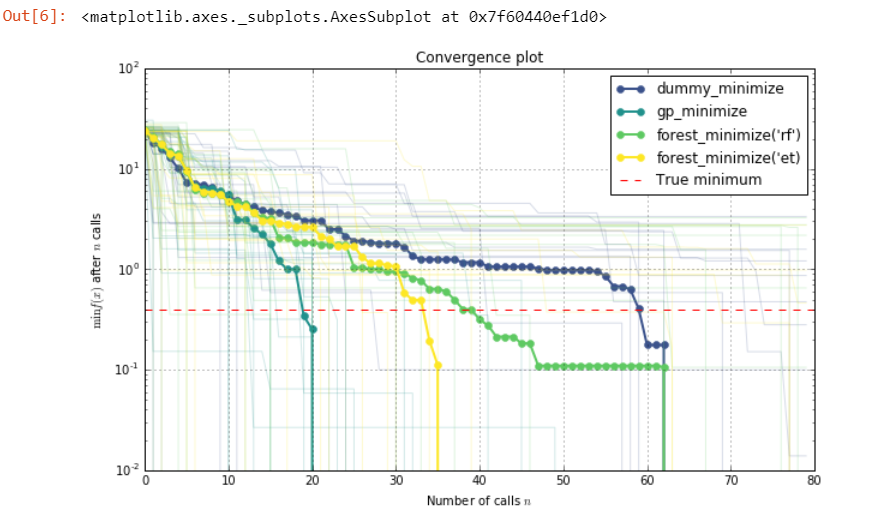top of page
Search

# Comparing Surrogate Models | Strategy Comparison With Bayesian Optimization or Sequential Model

Import Libraries And Generate Seeds

```import numpy as np
np.random.seed(123)

%matplotlib inline
import matplotlib.pyplot as plt
plt.rcParams["figure.figsize"] = (10, 6)
plt.set_cmap("viridis")```

Bayesian optimization or sequential model-based optimization uses a surrogate model to model the expensive to evaluate function func. There are several choices for what kind of surrogate model to use. This example compares the performance of:

• gaussian processes,

• extra trees, and

• random forests

as surrogate models. A purely random optimization strategy is used as a baseline.

Toy model

We will use the branin function as toy model for the expensive function. In a real world application this function would be unknown and expensive to evaluate.

```from skopt.benchmarks import branin as _branin

def branin(x, noise_level=0.):
return _branin(x) + noise_level * np.random.randn()```
```from matplotlib.colors import LogNorm

def plot_branin():
fig, ax = plt.subplots()

x1_values = np.linspace(-5, 10, 100)
x2_values = np.linspace(0, 15, 100)
x_ax, y_ax = np.meshgrid(x1_values, x2_values)
vals = np.c_[x_ax.ravel(), y_ax.ravel()]
fx = np.reshape([branin(val) for val in vals], (100, 100))

cm = ax.pcolormesh(x_ax, y_ax, fx,
norm=LogNorm(vmin=fx.min(),
vmax=fx.max()))

minima = np.array([[-np.pi, 12.275], [+np.pi, 2.275], [9.42478, 2.475]])
ax.plot(minima[:, 0], minima[:, 1], "r.", markersize=14, lw=0, label="Minima")

cb = fig.colorbar(cm)
cb.set_label("f(x)")

ax.legend(loc="best", numpoints=1)

ax.set_xlabel("X1")
ax.set_xlim([-5, 10])
ax.set_ylabel("X2")
ax.set_ylim([0, 15])

plot_branin()

```

Output:This shows the value of the two-dimensional branin function and the three minima.

Objective

The objective of this example is to find one of these minima in as few iterations as possible. One iteration is defined as one call to the branin function.

We will evaluate each model several times using a different seed for the random number generator. Then compare the average performance of these models. This makes the comparison more robust against models that get "lucky".

```
from functools import partial
from skopt import gp_minimize, forest_minimize, dummy_minimize

func = partial(branin, noise_level=2.0)
bounds = [(-5.0, 10.0), (0.0, 15.0)]
x0 = [2.5, 7.5]
n_calls = 80```

```def run(minimizer, n_iter=20):
return [minimizer(func, bounds, x0=x0, n_calls=n_calls, random_state=n)
for n in range(n_iter)]

# Random search
dummy_res = run(dummy_minimize)

# Gaussian processes
gp_res = run(gp_minimize)

# Random forest
rf_res = run(partial(forest_minimize, base_estimator="rf"))

# Extra trees
et_res = run(partial(forest_minimize, base_estimator="et"))```

Note that this can take a few minutes.

```from skopt.plots import plot_convergence

plot_convergence(("dummy_minimize", dummy_res),
("gp_minimize", gp_res),
("forest_minimize('rf')", rf_res),
("forest_minimize('et)", et_res),
true_minimum=0.397887, yscale="log")

```

Convergence PlotThis plot shows the value of the minimum found (y axis) as a function of the number of iterations performed so far (x axis). The dashed red line indicates the true value of the minimum of the branin function.

For the first ten iterations all methods perform equally well as they all start by creating ten random samples before fitting their respective model for the first time. After iteration ten the next point at which to evaluate branin is guided by the model, which is where differences start to appear.

Each minimizer only has access to noisy observations of the objective function, so as time passes (more iterations) it will start observing values that are below the true value simply because they are fluctuations.